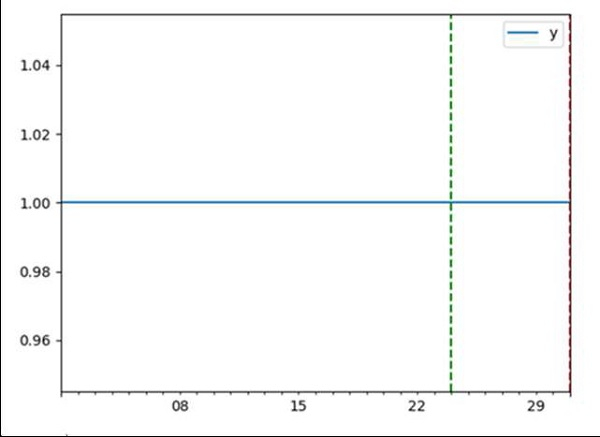# How do you plot a vertical line on a time series plot in Pandas?

Using Pandas, we will create a dataframe and set the vertical lines on the created axes, using axvline lines.

## Steps

• Using panda we can create a data frame.

• Creating a data frame would help to create help.

• Using axvline(), add a vertical line across the axes, where color is green, linestyle="dashed".

• Using axvline(), add a vertical line across the axes, where color is red, linestyle="dashed".

• Using plt.show(), show the plot.

## Example

import pandas as pd
from matplotlib import pyplot as plt
df = pd.DataFrame(index=pd.date_range("2019-07-01", "2019-07-31"))
df["y"] = 1
ax = df.plot()
ax.axvline("2019-07-24", color="green", linestyle="dashed")
ax.axvline("2019-07-31", color="red", linestyle="dashed")
plt.show()

## Output